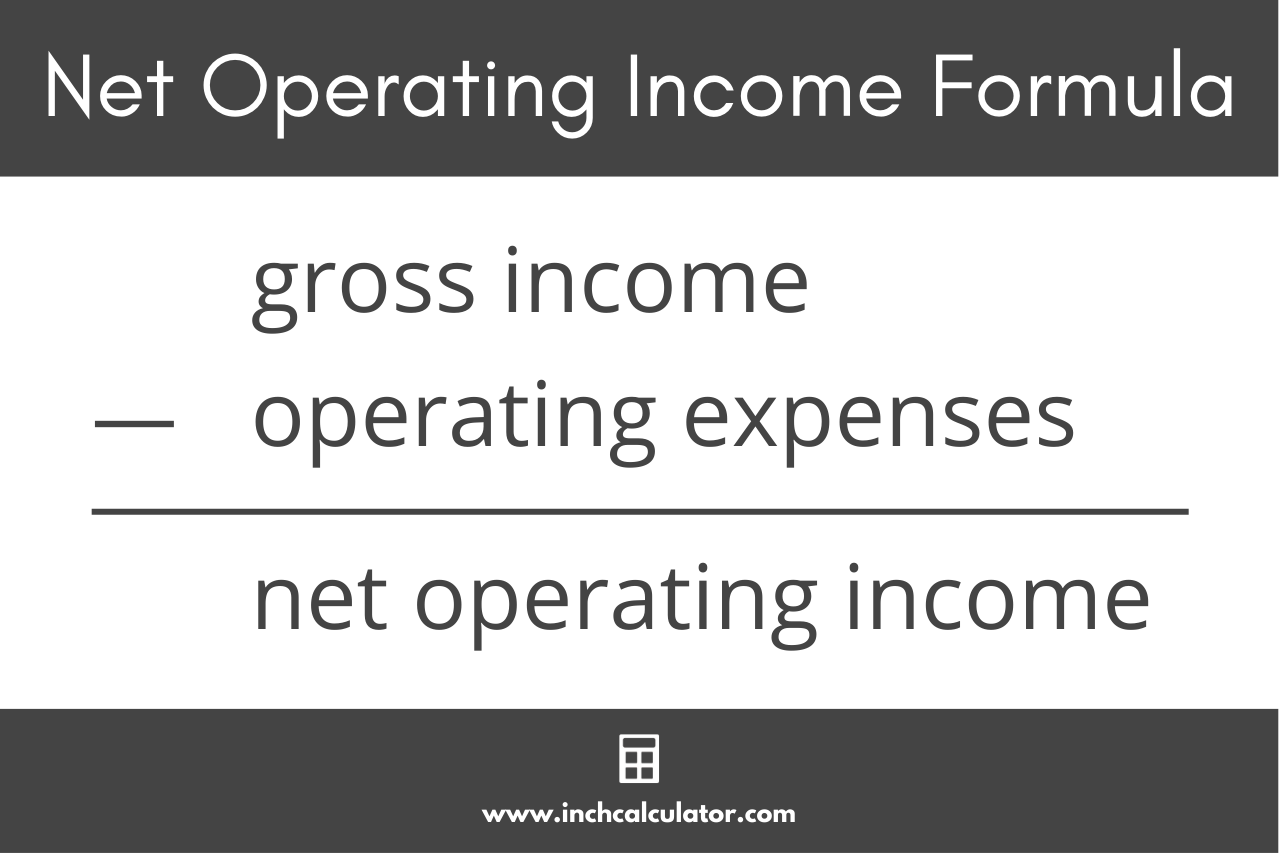# Net Operating Income Calculator

Use our net operating income calculator to calculate the NOI for an investment property below.

Income
\$
\$
Expenses
\$
\$
\$
\$
\$
\$
Optionally enter the property value to calculate the cap rate and gross rent multiplier
\$

## Results:

 Gross Income: \$ Operating Expenses: \$ Net Operating Income: \$ Cap Rate: Gross Rent Multiplier:
Learn how we calculated this below

## How to Calculate Net Operating Income

Net operating income (NOI) is a metric used in real estate to determine the profitability of an investment property. It is often used to evaluate whether the potential property is a viable investment opportunity.

NOI is one of various essential metrics used to evaluate the profitability of a property. It’s also used to generate other metrics, such as the cap rate and the gross rent multiplier for a property.

You might also be interested in using our cash on cash return calculator to evaluate the profitability of a property.

NOI is the net income the property generates after accounting for operating expenses. You can calculate it in a few easy steps.

### Step One: Calculate the Gross Income

Gross income is the total income generated by a property in a year, assuming it is fully leased or rented. The gross income is equal to the monthly rent multiplied by 12 (months per year).

Be sure to add any additional income, such as pet rent, parking space rentals, etc, to the rental income to find the total gross annual income.

### Step Two: Account for Vacancy and Credit Loss

Vacancy loss is the estimated loss due to a rental unit being unoccupied for a period of time. Credit loss is the loss due to a tenant not paying rent.

To estimate these losses, you need to determine the vacancy rate and credit loss rate. These will be approximations based on estimates for comparable properties in the area.

vacancy and credit loss = gross income × vacancy and credit loss rate

### Step Three: Calculate Operating Expenses

Operating expenses are expenses incurred to own, operate, and maintain the property. These include property taxes, insurance, utilities, maintenance, repairs, and property management fees.

To calculate the total operating expenses, simply add up all of these costs.

operating expenses = property taxes + insurance + utilities + maintenance + property management fees

### Step Four: Calculate Net Operating Income

Net operating income is the income generated by the property after accounting for vacancy and credit loss, and operating expenses. You can calculate NOI using the formula below.

#### Net Operating Income Formula

net operating income = gross income – vacancy & credit loss – operating expenses

Thus, the net operating income of the property is equal to the gross income minus the vacancy and credit loss minus the operating expenses.For example, let’s calculate the net operating income for a property that is expected to generate \$1,500 per month in rent and has total operating expenses of \$3,000 per year in taxes and management fees.

Let’s start by calculating the gross annual income by multiplying the rent by 12.

gross annual income = \$1,500 × 12 = \$18,000

Then, subtract the operating expenses.

net operating income = \$18,000 – \$3,000 = \$15,000

So, in this example, the property has a net operating income of \$15,000 per year. This does not take into consideration the vacancy loss or credit loss, which may or may not be applicable in a given year.